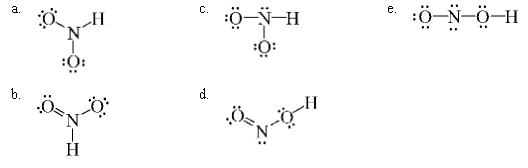# Problem: Which of the following is a correct Lewis structure for nitrous acid, HNO 2?

###### FREE Expert Solution

Draw the Lewis structure of nitrous acid, HNO2 using the concept of octet rule

To do so, we first need to do the following steps:

Step 1: Determine the central atom in this molecule.

Step 2: Calculate the total number of valence electrons present.

Step 3: Draw the Lewis structure for the molecule

86% (269 ratings)###### Problem Details

Which of the following is a correct Lewis structure for nitrous acid, HNO 2?